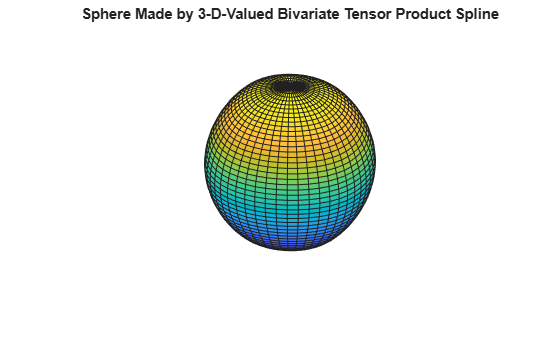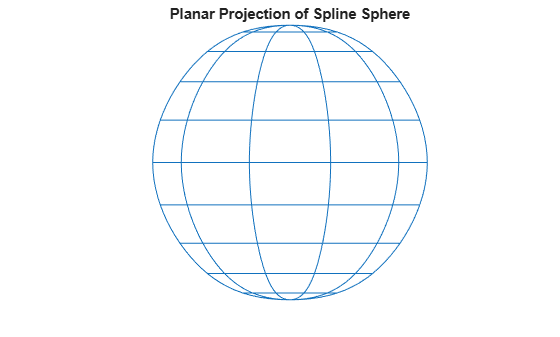# Fitting Values at N-D Grid with Tensor-Product Splines

Vector-valued splines are also used in the approximation to gridded data, in any number of variables, using tensor-product splines. The same spline-construction commands are used, only the form of the input differs. For example, if `x` is an `m`-vector, `y` is an `n`-vector, and `z` is an array of size `[m,n]`, then `cs = csapi({x,y},z);` describes a bicubic spline f satisfying $f\left(x\left(i\right),y\left(j\right)\right)=z\left(i,j\right)$ for $i=1:m,j=1:n$. Such a multivariate spline can be vector-valued. For example, the following gives a perfectly acceptable sphere.

```x = 0:4; y = -2:2; s2 = 1/sqrt(2); z(3,:,:) = [0 1 s2 0 -s2 -1 0].'*[1 1 1 1 1]; z(2,:,:) = [1 0 s2 1 s2 0 -1].'*[0 1 0 -1 0]; z(1,:,:) = [1 0 s2 1 s2 0 -1].'*[1 0 -1 0 1]; sph = csape({x,y},z,["clamped","periodic"]); fnplt(sph) axis equal axis off title("Sphere Made by 3-D-Valued " + ... "Bivariate Tensor Product Spline")```The sphere's projection onto the (x,z)-plane is plotted by the following.

```fnplt(fncmb(sph,[1 0 0; 0 0 1])) axis equal axis off title("Planar Projection of Spline Sphere")```